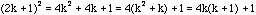Date: Thu, 26 Nov 1998 21:00:05 -0400 Hi Pick any odd number, square it, and then divide it by 8. No matter what odd number is chosen and squared and divided by 8, the remainder is one. Could you please explain this to me or is there a pattern that I am not aware of Respectfully Submitted Brenda Hi again Brenda, Since the number you start with is odd you can write it 2k+1, that is, it is one more than an even number. Squaring 2k+1 givesThus the square of 2k+1 is one more than 4k(k+1). Since k and k+1 are consecutive integers one of them must be even, and hence 2 divides k(k+1). Thus 8 divides 4k(k+1) and hence 4k(k+1)+1 is one more than a multiple of 8. That is dividing 4k(k+1)+1 by 8 leaves a remainder of 1. Cheers, Harley Go to Math Central To return to the previous page use your browser's back button.